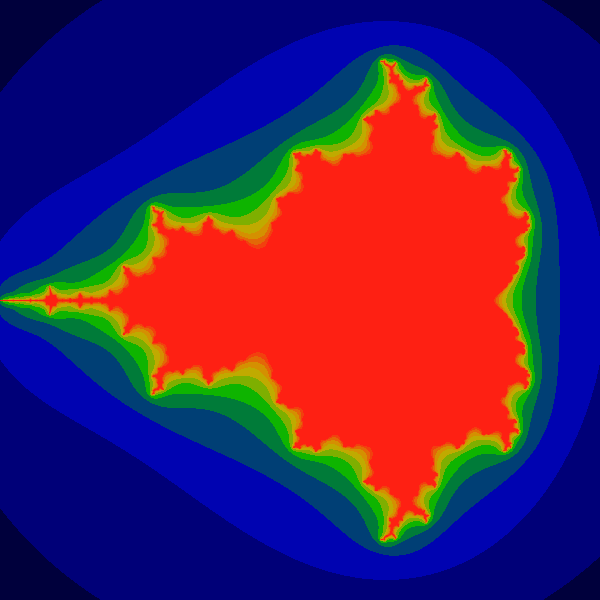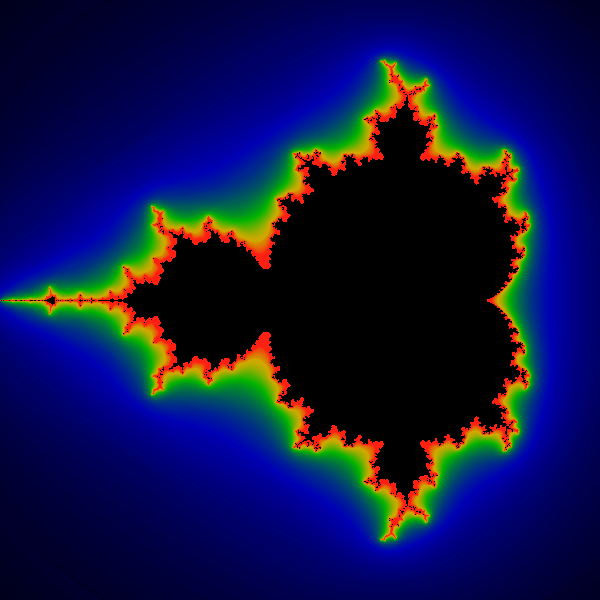# Smooth Shading for the Mandelbrot Exterior

Q: How can I smoothly shade the exterior of the Mandelbrot set?

A: Most pictures of the exterior of the Mandelbrot set use the "escape count" of the iteration. The "escape count" is the number of times that the iteration is performed until the iterated value exceeds some radius. Normally, this count is an integer, and thus, when it is used to select a color, "bands" (areas of constant color) appear.

Banding can be eliminated by using a fractional escape count. There are many ways that a fractional escape count can be computed. One possible way of obtaining a fraction is to measure how far the iterated point landed outside of th escape cutoff. One of the smoothest, most mathematically correct formulas is the "renormalized" iteration count.

For the iterated equation z(n+1) = z(n) ^ 2 + c, the renormalized count is given by

```mu = N + 1 - log (log  |Z(N)|) / log 2
```

where N is the usual "escape count", Z(N) is the value of Z when it escaped, and |z| is the modulus of z. (that is, if z=x+iy then |z| = sqrt (x*x+y*y) ).

For the more general formula z(n+1) = z(n) ^ k + c, the renormalized count is

```mu = N + 1 - log (log  |Z(N)|) / log k
```

Although it is not obvious from the formula, the value of mu is almost completely independent of the actual escape radius. This is because the mathematics used to derive the formula implicitly take the limit of the escape radius going to infinity, and then subtracting the infinite iteration count resulting from taking that limit. (This formula shows only the first term).

The following simplified and very insightful derivation of the formula is provided by Earl L. Hinrichs:

"Ignoring the +C in the usual formula, the orbit point grows by Z := Z^2. So we have the progression Z, Z^2, Z^4, Z^8, Z^16, etc. Iteration counting amounts to assigning an integer to these values. Ignoring a multiplier, the log of this sequence is: 1, 2, 4, 8, 16. The log-log is 0, 1, 2, 3, 4 which matches the integer value from the iteration counting. So the appropriate generalization of the discrete iteration counting to a continuous function would be the double log."

## References:

http://linas.org/art-gallery/escape/smooth.html

The theory plus algorithms and examples:
http://linas.org/art-gallery/escape/escape.html

Some of the Mathematics behind the Theory:
http://linas.org/art-gallery/escape/math.html

## Sample Image

The two pictures below show, respectively, the typical form of banding as typically seen when an integer iteration count is used, and the same calculation, with colors selected by using the renormalized iteration count.Radius of 3.1. The above shows the the typical banding resulting from the use of the iteration count to choose colors. This image was generated with a maxiter=18 and an escape radius of three.Radius of 3.1. The above shows the disappearance of bands when the renormalized, fractional iteration count is used to select colors. The iteration parameters are the same as above: maxiter=18 and an escape radius of three.

Created by Linas Vepstas 21 October 1997
linas@linas.org